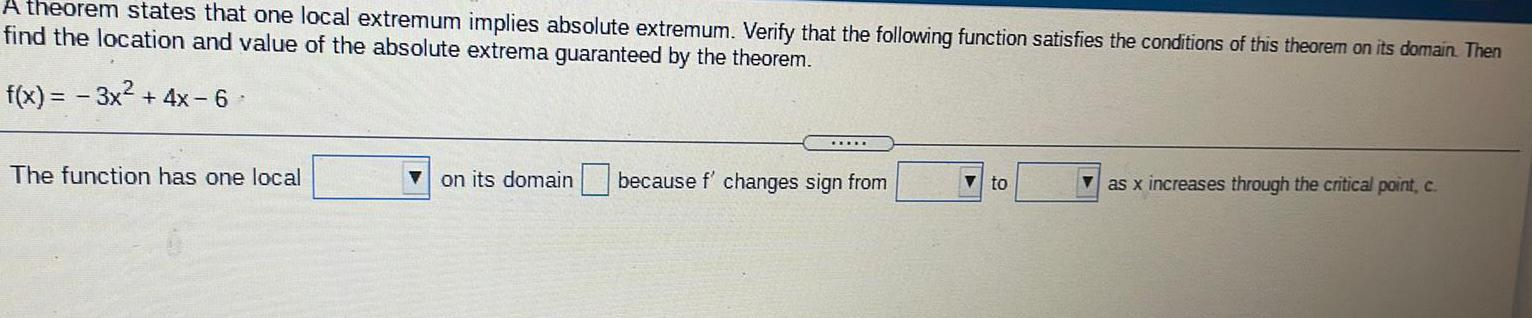Question:

# A theorem states that one local extremum implies absoluteA theorem states that one local extremum implies absolute extremum. Verify that the following function satisfies the conditions of this theorem on its domain. Then find the location and value of the absolute extrema guaranteed by the theorem. f(x) = -3x² + 4x-6 The function has one local__ on its domain __ because f' changes sign from __to __ as x increases through the critical point, c.